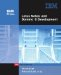# Real-World Examples Using the Formula Language

By Steve Kern

IN THIS CHAPTER

• Programming Practices
• Using Formulas in Forms and Subforms
• Writing Field Formulas
• Writing View Formulas
• Using Hide When Formulas
• Working with Forms, Views, and Shared Actions

In this chapter, you will learn about the various places where you can write formulas, as well as how to write those formulas. In Chapter 12, "Using the Formula Language," you learned about the components of the Formula language and its syntax. You learned about types of formulas and how to work with the various types of @Functions. This chapter extends that knowledge into specific areas where you can use the Formula language in the real world.Lotus Notes and Domino 6 Development (2nd Edition)
ISBN: 0672325020
EAN: 2147483647
Year: 2005
Pages: 288